# Algebra - math word problems

#### Number of problems found: 2654

• Acid evaporationHow many kilograms of water do we have to evaporate from 100 kg of 32% acid to make it 80% concentration?
• LegsCancer has 5 pairs of legs. The insect has 6 legs. 60 animals have a total of 500 legs. How much more are cancers than insects?
• Father and daughterWhen I was 11 my father was 35 year old. Now, father has three times older then me. How old is she?
• Eggs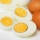3400 eggs sliced hens for February and March. We have to calculate how many hens can make 3400 eggs when one hen give 2 eggs a day for 59 days.
• Granules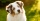Katy had two dogs. The first one ate a pack of granules a week and the other two times longer. Katka bought them two bags of granules. How long does it last for both dogs?
• Journey 5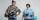A man has to do a journey of 84km in 3 hours. He travels the first 30km at 20km/hr. At what rate must he travel the remaining distance to complete his journey on time?
• Angle at the apex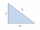In an isosceles triangle, the angle at the apex is 30° greater than the angle at the base. How big are the internal angles?
• Three paintersOne painter would have painted the fence for 15 hours, the second in 12 hours. The plot had to be painted in four hours, so they called the third one, and all worked together. For what time would the third painter paint fence the fence alone?
• Triangle perimeterCalculate the triangle perimeter whose sides are in ratio 3: 5: 7 and the longest side is 17.5 cm long.
• PupilsThere are 350 girls in the school, and the other 30% of the total number of pupils are boys. How many pupils does the school have?
• Equation - inverseSolve for x: 7: x = 14: 1000
• Reminder and quotientThere are given the number C = 281, D = 201. Find the highest natural number S so that the C:S, D:S are with the remainder of 1,
• Reminder and quotientThere are given numbers A = 135, B = 315. Find the smallest natural number R greater than 1 so that the proportions R:A, R:B are with the remainder 1.
• Collection of stampsJano, Rado, and Fero have created a collection of stamps in a ratio of 5: 6: 9. Two of them had 429 stamps together. How many stamps did their shared collection have?
• Mushrooms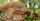Grandfather gathered fresh mushrooms. The fifth was wormwood, and it was thrown away, the other dried up. He obtained 720 grams of dried mushrooms. How many kilograms did the grandfather collect, and by drying the mushrooms they lost 75% of their weight?
• Two pipesHow long will the pool be filled with a double supply pipe if it takes the pool to fill the first pipe by 4 hours longer and the second pipe 9 hours longer than both pipes open at the same time?
• InqualitySolve inequality: 3x + 6 > 14
• Two citiesThe car goes from city A to city B at an average speed of 70 km/h, back at an average speed of 50 km/h. If it goes to B and back at an average speed of 60 km/h, the whole ride would take 8 minutes less. What is the distance between cities A and B?
• Simple equation 6Solve equation with one variable: X/2+X/3+X/4=X+4
• Find unknownFind unknown numerator: 4/8 + _/8 = 1

Do you have an interesting mathematical word problem that you can't solve it? Submit a math problem, and we can try to solve it.

We will send a solution to your e-mail address. Solved examples are also published here. Please enter the e-mail correctly and check whether you don't have a full mailbox.

Please do not submit problems from current active competitions such as Mathematical Olympiad, correspondence seminars etc...